# 显示支撑/阻力位

19 二月 2016, 11:100
2 880

### 简介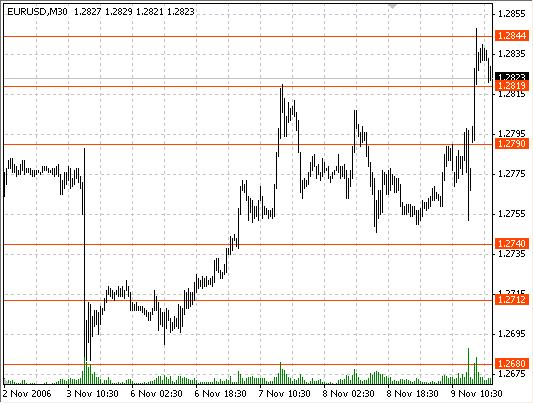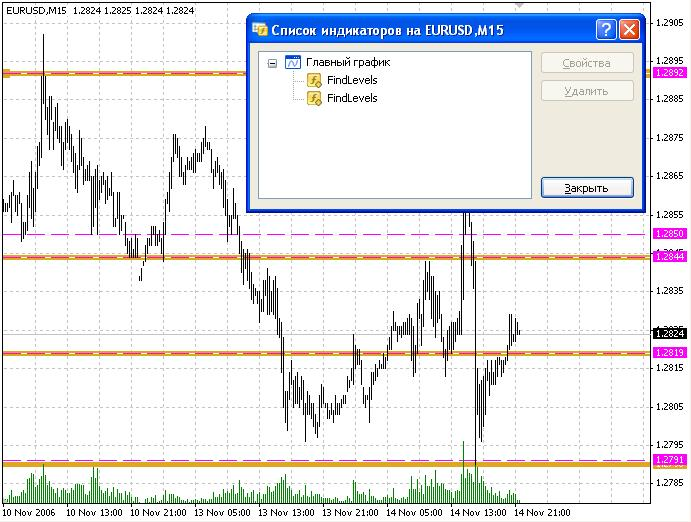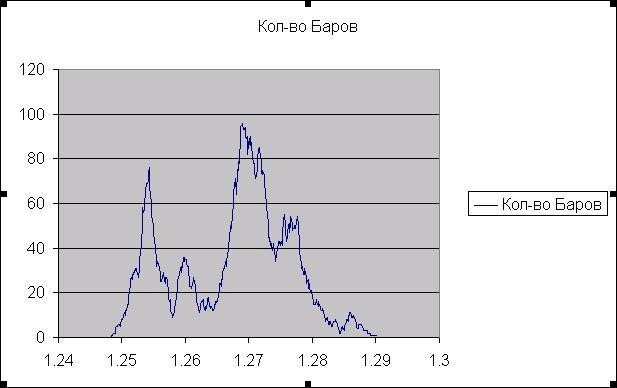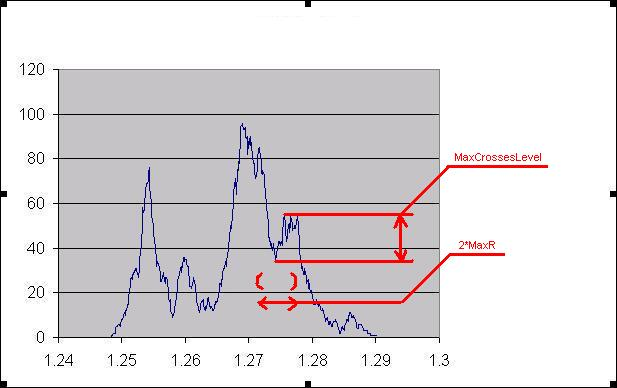### 辅助函数

```double prLow(int i)
{
return (iLow(NULL,TimePeriod,i));
}

double prHigh(int i)
{
return (iHigh(NULL,TimePeriod,i));
}

```

```int Period2Int(int TmPeriod)
{
switch(TmPeriod)
{
case PERIOD_M1  : return(0);
case PERIOD_M5  : return(1);
case PERIOD_M15 : return(2);
case PERIOD_M30 : return(3);
case PERIOD_H1  : return(4);
case PERIOD_H4  : return(5);
case PERIOD_D1  : return(6);
case PERIOD_W1  : return(7);
case PERIOD_MN1 : return(8);
}
return (0);
}

string Period2AlpthabetString(int TmPeriod)
{
return(Alphabet[Period2Int(TmPeriod)]);
}```

### 编写指标

```extern int MaxLimit = 1000;
extern int MaxCrossesLevel = 10;
extern double MaxR = 0.001;
extern int TimePeriod = 0;
extern color LineColor = White;
extern int LineWidth = 0;
extern int LineStyle = 0;```
• MaxLimit - 所使用的历史柱的数量；
• MaxCrossesLevel – 局部最大值和局部最小值的最小差异（关于详细描述请参阅“理论综述”一节；
• MaxR – 检出的最小值所在区域的半径；
• TimePeriod – 用于检测支撑位的时间范围； 默认为映射窗口的时间范围；
• LineColor – 显示的线条颜色；
• LineWidth – 线条宽度，默认为 0；
• LineStyle – 线型，默认为 0。

```int init()
{
if(TimePeriod == 0)
TimePeriod = Period();

if(TimePeriod != 0 && LineWidth == 0)
if(Period2Int(TimePeriod) - Period2Int(Period()) >= 0)
LineWidth = Widths[Period2Int(TimePeriod) - Period2Int(Period())];
else
{
LineWidth = 0;
if(LineStyle == 0)
LineStyle = STYLE_DASH;
}

if(TimePeriod != 0 && LineColor == White)
LineColor = Colors[Period2Int(TimePeriod)];

return(0);
}```

Colors[] 和 Width[] 数组定义方式如下：

```color  Colors[] = {Red,Maroon, Sienna, OrangeRed, Purple,I ndigo,
DarkViolet, MediumBlue, DarkSlateGray};
int    Widths[] = {1, 2, 3, 4, 5, 6, 7, 8, 9};
```

```int CrossBarsNum[];
bool CrossBarsMin[];
double d1Num = 0.0, d2Num = 0.0;
datetime TMaxI = 0;```
• CrossBarsNum[] 数组 – 为每个价格指示柱的数量的数组；
• CrossBarsMin[] – 跟设定价格线是否为局部最小值相对应的数组；
• d1Num 和 d2Num – 属于从 0 到 MaxLimit 柱区间的最低价格和最高价格；
• TMaxI – 指示最后处理柱的时间。
```#define MaxLines 1000
string LineName[MaxLines];
int LineIndex = 0;```
• MaxLines - 创建线条的最大数量；

• LineName[] – 线条名称的数组；

• LineIndex – LineName[] 数组中空单元的索引。

```int counted_bars = IndicatorCounted();
int limit = MathMin(Bars - counted_bars, MaxLimit);

double d1 = prLow(iLowest(NULL, TimePeriod, MODE_LOW, limit, 0));
double d2 = prHigh(iHighest(NULL, TimePeriod, MODE_HIGH, limit, 0));```

```if(d1Num != d1 || d2Num != d2)
{
ArrayResize(CrossBarsNum, (d2 - d1)*10000);
ArrayResize(CrossBarsMin, (d2 - d1)*10000);

if(d1Num != d1 && d1Num != 0.0)
{
ArrayCopy(CrossBarsNum, CrossBarsNum, 0, (d1Num - d1)*10000);
ArrayCopy(CrossBarsMin, CrossBarsMin, 0, (d1Num - d1)*10000);
}

d1Num = d1;
d2Num = d2;
}```

```for(double d = d1; d <= d2; d += 0.0001)
{
int di = (d - d1)*10000;
for(int i = 1; i < limit; i++)
if(d > prLow(i) && d < prHigh(i))
CrossBarsNum[di]++;
if(Time[limit] != TMaxI&&TMaxI != 0)
if(d > prLow(iBarShift(NULL, 0, TMaxI)) &&
d < prHigh(iBarShift(NULL, 0, TMaxI)))
CrossBarsNum[di]--;
}
TMaxI = Time[limit] - 1;```

```double l = MaxR*10000;
for(d = d1 + MaxR; d <= d2 - MaxR; d += 0.0001)
{
di = (d - d1)*10000;
if(!CrossBarsMin[di] && CrossBarsNum[ArrayMaximum(CrossBarsNum, 2*l, di - l)] -
CrossBarsNum[ArrayMinimum(CrossBarsNum, 2*l, di - l)] > MaxCrossesLevel &&
CrossBarsNum[di] == CrossBarsNum[ArrayMinimum(CrossBarsNum, 2*l, di - l)] &&
CrossBarsNum[di-1] != CrossBarsNum[ArrayMinimum(CrossBarsNum, 2*l, di - l)])
{
CrossBarsMin[di] = true;
LineName[LineIndex] = Period2AlpthabetString(TimePeriod) + TimePeriod + "_" + d;
ObjectCreate(LineName[LineIndex], OBJ_HLINE, 0, 0, d);
ObjectSet(LineName[LineIndex], OBJPROP_COLOR, LineColor);
ObjectSet(LineName[LineIndex], OBJPROP_WIDTH, LineWidth);
ObjectSet(LineName[LineIndex], OBJPROP_STYLE, LineStyle);
LineIndex++;
}
if(CrossBarsMin[di] && CrossBarsNum[di] !=
CrossBarsNum[ArrayMinimum(CrossBarsNum, 2*l, di - l)])
{
CrossBarsMin[di] = false;
ObjectDelete(Period2AlpthabetString(TimePeriod) + TimePeriod + "_" + d);
}
}```

```CrossBarsNum[di] == CrossBarsNum[ArrayMinimum(CrossBarsNum, 2*l, di - l)] &&
CrossBarsNum[di-1]!= CrossBarsNum[ArrayMinimum(CrossBarsNum, 2*l, di - l)]```

### 指标互相作用

1. 应能多次运行指标，且指标应具有时间期间内的输入数据；

2. 线条应不同，要容易区分每个支撑位所属的时段；

3. 所有线条都应该长期和短期跟踪。

```string Period2AlpthabetString(int TmPeriod)
{
return (Alphabet[Period2Int(TmPeriod)]);
}```
Alphabet 是逆序拉丁字母的数组。 每个支撑位的全名如下： Period2AlpthabetString(TimePeriod)+TimePeriod+"_"+d。### 总结

FindLevels.mq4 (5.21 KB)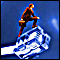绘制支撑/阻力位的方法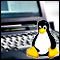使用 Linux 交易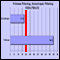按记录过滤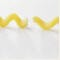测试程序中的断点：这是可能的！